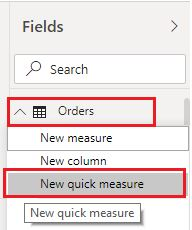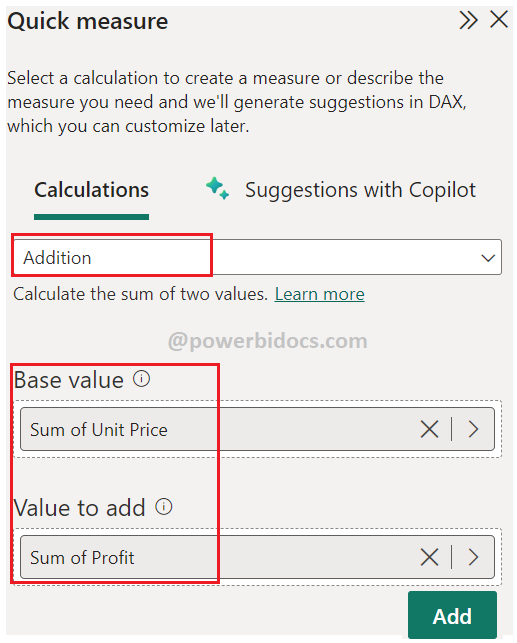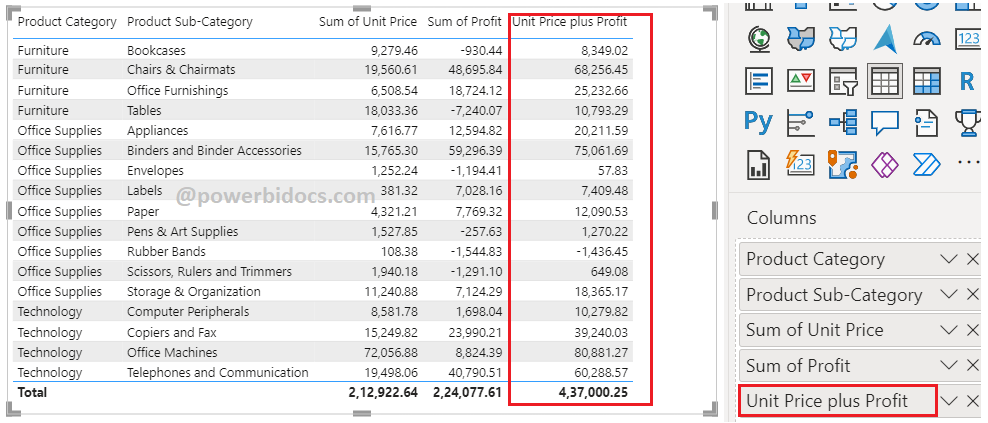Home » Power BI » Power BI – Quick Measures

# Power BI – Quick MeasuresQuick measures are pre-built or ready-made measures. You don’t have to write a DAX formula for them; it’s done for you based on the input you provide in a quick measure dialog box.

Step-1: Open the Power BI file and load the sample dataset.

Step-2: Right-click on the ‘Orders’ dataset, then click on ‘New quick measure.’Quick Measure

Step-3: After that, the Quick Measure dialog box appears, allowing you to select your desired calculations.Quick measure options PBI

### Quick measures provide calculations in six categories, which are:

• Aggregate per category
• Filters
• Time intelligence
• Totals
• Mathematical operations
• Text

So, let’s begin with Mathematical operations. Below is a screenshot of the sample dataset.Sample data set

Now, add the ‘Unit Price’ and ‘Profit’ fields using the quick measure for addition calculation.

Drag the ‘Profit’ and ‘Unit Price’ fields into the ‘Base value’ box and the ‘Value to add’ box, then click the ‘Add’ button to proceed further.Quick measure base and value options

#### Created measure by Quick Measures as below

```Unit Price plus Profit =

SUM(‘Orders'[Unit Price]) + SUM(‘Orders'[Profit])```

Now, drag the new measure into the table visual, and it will return the sum of Profit and Unit Price in a new column.Quick Measure output

You can follow the same process to work with other calculations.

Similar Post: Quick measure suggestions in Power BI

Hope you enjoyed the post. Your valuable feedback, question, or comments about this post are always welcome or you can leave us message on our , we will revert to you asap.